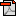[Week 2] Programming with R

If

기본적인 제어문이다.

if(<condition>) {

## do something

} else {

## do something else

}

if(<condition1>) {

## do something

} else if(<condition2>) {

## do something different

} else {

## do something different

}

else는 없어도 된다.for / while

for(i in 1:10) {

print(i)

}

This loop takes the i variable and in each iteration of the loop gives it values 1,2,3, ... , 10, and then exits.

These three loops have the same behavior.

x <- c("a", "b", "c", "d")

for(i in 1:4) {

print(x[i])

}

for(i in seq_along(x)) {

print(x[i])

}

for(letter in x) {

print(letter)

}

for(i in 1:4) print(x[i])

z <- 5

while(z >= 3 && z <= 10) {

print(z)

coin <- rbinom(1, 1, 0.5)

if(coin == 1) { ## random walk

z <- z + 1

} else {

z <- z - 1

}

}

Repeat

break를 써야만 중단할 수 있는 무한 루프를 생성 하게 된다.

The loop in the previous slide is a bit dangerous because there's no guarantee it will stop.

Better to set a hard limit on the number of iterations (e.g. using a for loop) and then report whether convergence was achieved or not.

Next

해당 iteration을 무시함.Return

만나는 즉시 해당 function에서 나오게 된다.

그리고 주어진 값을 반환 한다.

Functions

기본적인 예제Functions are created using the function() directive and are stored as R objects just like anything else.

In particular, they are R objects of class "function".

f <- function (<arguments>) {

## Do something interesting

}

Functions in R are "first class objects", which means that they can be treated much like any other R object.

Importantly,

Functions can be passed as arguments to other functions

Functions can be nested, so that you can define a function inside of another function The return value of a function is the last expression in the function body to be evaluated.

Argument Matching

You can mix positional matching with matching by name. When an argument is matched by name, it is "taken out" of the argument list and the remaining unnamed arguments are atched in the order that they are listed in the function definition.

Most of the time, named arguments are useful on the command line when you have a long argument list and you want to use the

Lazy Evaluation

Arguments to functions are evaluated lazily, so they are evaluated only as needed.

f<- function (a,b)

{ a^2 }

f(2)

두번째 인자를 사용하지 않아도 에러가 발생하지 않는다.

실제로 b를 사용하지 않기 때문이다.

f <- function (a,b) {

print (a)

print (b)

}

f(45)

 45

Error: Argument "b" is missing, with no default

lazy evaluation의 의미는 위에서 보는것과 같이 최대한 error check을 뒤로 미뤄서 한다는 것에 있다.

The "..." Argument

The ... argument indicate a variable number of arguments that are usually passed on to other functions.

... is often used when extending another function and you don't want to copy the entire argument list of the original function.

myplot <- function (x, y, type = "1", ...) {

plot(x, y, type = type, ...)

}

Generic functions use ... so that extra arguments can be passed to methods (more on this later).

> mean

function (x, ...)

UseMethod("mean")

Scoping Rules

The scoping rules for R are the main feature that make it different from the original S language.

The scoping rules determine how a value is associated with a free variable in a function

R uses lexical scoping or static scoping. A common alternative is dynamic scoping.

Related to the scoping rules is how R uses the search list to bind a value to a symbol

Lexical scoping turns out to be particularly useful for simplifying statistical computations

Free variable의 정의

이것은 formal argument 가 아니다.

이것은 local variable이 아니다.

Lexical ScopingExploring a Function ClosureLexical vs. Dynamic Scoping함수 f에서 y와 함수 g는 free 변수 이다.

함수 G에서는 y가 free variable 이다.

With lexical scoping the value of y in the function g is looked up in the environment in which the function was defined,

in this case the global environment, so the value of y is 10.

With dynamic scoping, the value of y is looked up in the environment from which the function was called

(sometimes referred to as the calling environment).

- In R the calling environment is known as the parent frame

So the value of y would be 2.

When a function is defined in the global environment and is subsequently called from the global environment, then the defining environment and the calling environment are the same. This can sometimes give the appearance of dynamic scoping.Coding Standards

1. Always use text files / text editor

3. Limit the width of your code (80 columns?)

4. Limit the length of individual functions

아래는 들여쓰기 공간을 4로 준것이고, 배경도 다크로 변경한 것이다.

[tool] -> [global options]Dates and Times in R

R has developed a special representation of dates and times

Dates are represented by the Date class

Times are represented by the POSIXct or the POSIXlt class

Dates are stored internally as the number of days since 1970-01-01

Times are stored internally as the number of seconds since 1970-01-01

There are a number of generic functions that work on dates and times

weekdays: give the day of the week

months: give the month name

quarters: give the quarter number (“Q1”, “Q2”, “Q3”, or “Q4”)Quiz 02

10문제이고 풀이는 첨부 파일과 같다.Quize 2 week (answer).pdf

Programming Assignment 1: Air Pollution

처음한 프로그래밍 과제

제출 방식이 R script를 이용한다.

아래와 같이 정상적으로 업로드 할경우 완료되는 것을 볼 수 있다.#### 'MOOC > R Programming' 카테고리의 다른 글

 Certificate & Comment  (0) 2015.08.26 2015.07.30 2015.07.24 2015.07.16 2015.07.08